# Solve Discontinuous Function Problems with Wolfram|Alpha

June 13, 2012 —Comments Off

It’s usually in precalculus class that students are first exposed to the more exotic and subtle aspects of functions on the real line. This first exposure comes through studying limits and discontinuities.

Most functions that we see every day, from the parabolic arc of a thrown ball to the exponential growth of money in a bank account, are “continuous.” That is, they don’t change their value suddenly. Thought of another way, a continuous function is one you can graph without having to lift your pen up from the paper.

Conversely, a discontinuity of a function is a point where the value of the function experiences a sudden change. In technical language, a discontinuity of a function from reals to reals is a point where either the left- or right-hand limit does not exist, or where these limits exist but aren’t both equal to the value of the function at this point.

Wolfram|Alpha now has the ability to find and analyze the discontinuities of most functions of real numbers. When it comes to such functions, there are three main kinds of discontinuities.

An “infinite” discontinuity is a point where the function increases to infinity and/or decreases to negative infinity (i.e., where it has a vertical asymptote). 1/x is the standard example: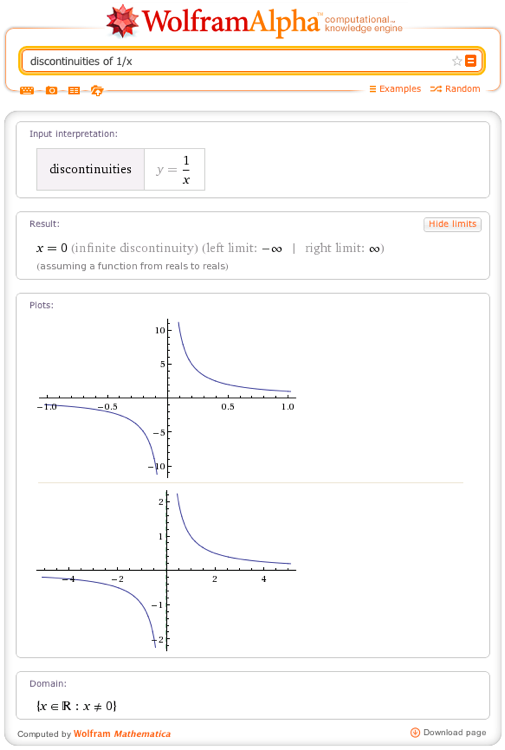A “jump” discontinuity is where the left- or right-hand limits are both real numbers (not infinity) but are not equal. This is epitomized by the “unit step” function (think of flipping on a light switch).

Besides finding the discontinuities, Wolfram|Alpha will attempt to compute the left- and right-hand limits at each discontinuity as well as the value of the function at the discontinuity, if it exists. Clicking the Show limits button reveals this information: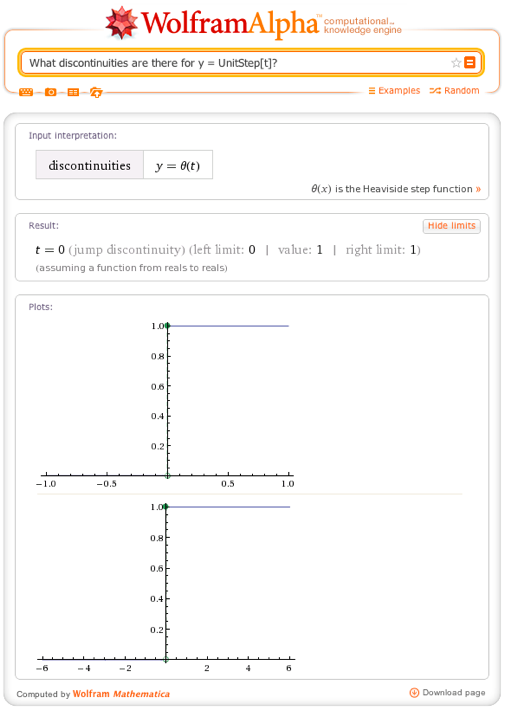The final type of discontinuity is called a “removable” discontinuity. This is where the left- or right-hand limits are both the same real number (not infinity), but not equal to the value of the function. A simple example is (x – 1) / (x – 1), which is equal to 1 everywhere except at x = 1, where it is undefined.

Here is a more complicated example: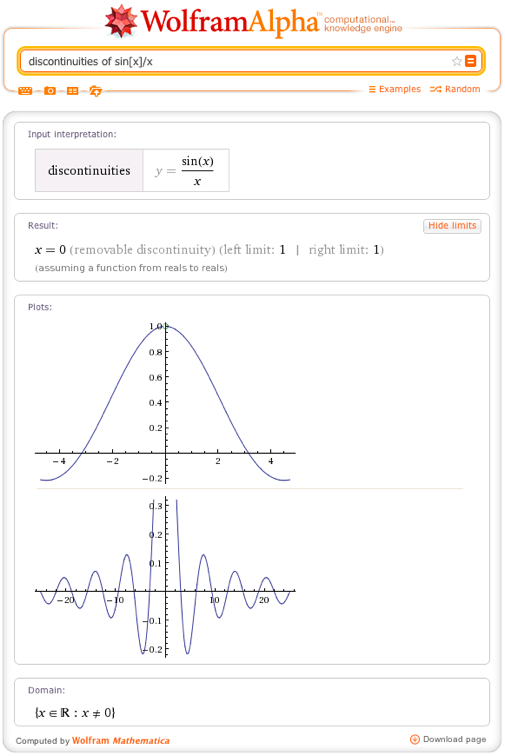We can think of “removing” a removable discontinuity by just defining a function that is equal to the limit at the point of discontinuity, and the same otherwise. If we do this with (x – 1) / (x – 1), we just get the constant function f(x) = 1. In the case of sin(x) / x, defining the value at x = 0 to be 1 (the value of the limit at x = 0) yields an important continuous function for communications engineering: the “sinc” function.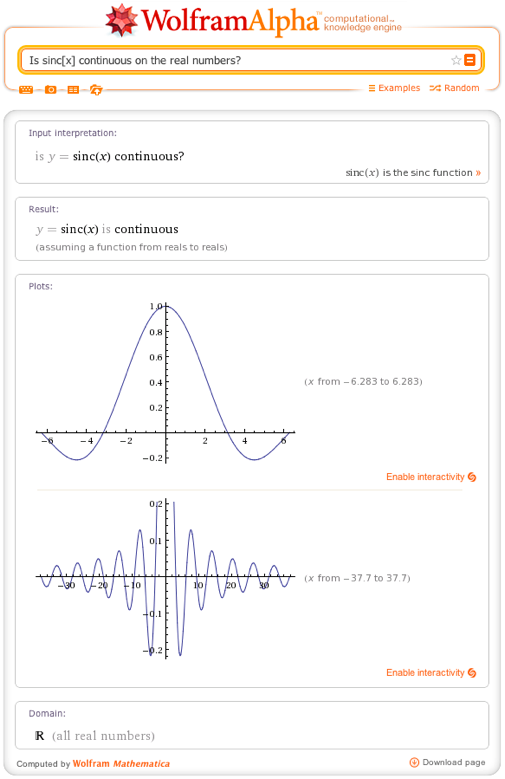A more exotic kind of discontinuity is found in the function sin[1/x]: at x = 0, the left- and right-hand limits do not exist and are also not infinite, because the function oscillates infinitely often between -1 and 1, a fact which Wolfram|Alpha (with help from Mathematica) is able to figure out.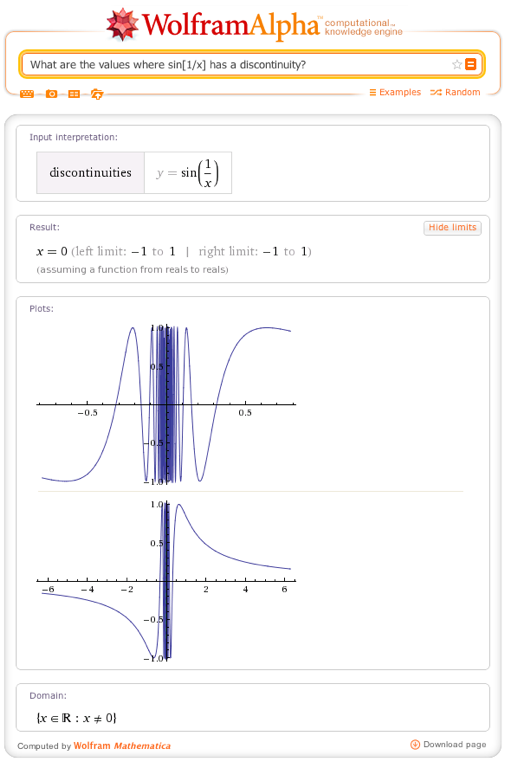The discontinuities of a parametric function are just where any of its component functions have a discontinuity: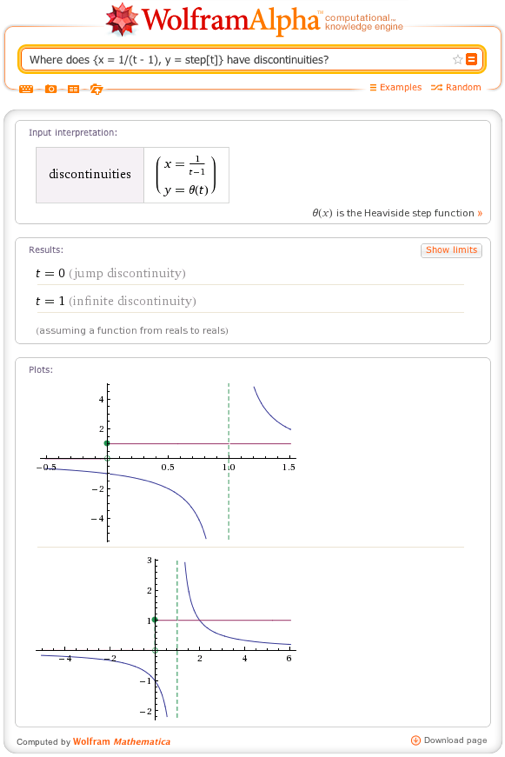Higher-dimensional functions also have discontinuities, of course, which can be infinitely more complicated than those on the real line. While Wolfram|Alpha can’t find and analyze all of them, it can analyze some other useful classes of them. For example, Wolfram|Alpha finds the “poles” of a function on the complex plane, giving their orders and residues: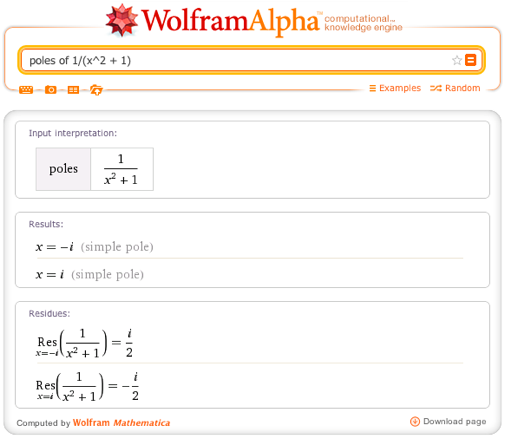This new ability to analyze discontinuities is part of our ongoing effort to make Wolfram|Alpha the best possible mathematical knowledge engine. As always, we look forward to reading your feedback and bringing you more fun and useful abilities in the future.

Much interesting.. Particularly interesting about higher-dimensional functions.

Posted by Vladislav June 15, 2012 at 3:19 am

Nice. It may have been nice to discuss the recursive nature of computing these discontinuities so people have an idea of how Alpha has magically computed these results…. This would be especially relevant as it relies almost entirely on symbolic methods so easily implemented in mathematica.

Posted by Sam Blake June 18, 2012 at 11:59 am

is the Differentiation of arcsin(2*x/(1+x^2)) is (2/(1+x^2)) for x is the element of [0,1] and -(2/(1+x^2)) for x is the element of [1, infinity] I have analyzed on the basis of the graph of the function ?

Posted by Vijay Kumar Agarwal July 3, 2012 at 12:22 pm

Intriguing to say the least

Posted by Pascal Roget July 2, 2013 at 7:13 am

So, does a discontinuous function become differentiable for all domains?

Posted by Nick May 21, 2014 at 12:49 pm

I am trying to make e to the exp dt with d=d {one} for tor =c with t being time into a continuous function. I don’t have the right keyboard to show this, but d {one} is one value of d, say .1, and d {two} is a second d value, say .2. Time t moves mechanically so the construction is parametric and is part of a calculus of variations maximizing problem that is in continuous time.

Posted by John Grow June 18, 2015 at 2:35 pm